Home | | Physics 12th Std | Electromagnetic Induction and Alternating Current: Questions and Answers

# Electromagnetic Induction and Alternating Current: Questions and Answers

Physics : Electromagnetic Induction and Alternating Current: Short Answer Questions, Long Answer Questions, Important Questions

1. What is meant by electromagnetic induction?

Whenever the magnetic flux linked with a closed coil changes, an emf (electromotive force) is induced and hence an electric current flows in the circuit. This current is called an induced current and the emf giving rise to such current is called an induced emf. This phenomenon is known as electromagnetic induction.

2. State FaradayŌĆÖs laws of electromagnetic induction.

First law:

Whenever magnetic flux linked with a closed circuit changes, an emf is induced in the circuit.

Second law:

The magnitude of induced emf in a closed circuit is equal to the time rate of change of magnetic flux linked with the circuit.

If the magnetic flux linked with the coil changes by dŽĢin a time dt, then the induced emf is given by

╬Ą = dŽĢB / dt3. State LenzŌĆÖs law.

Lenz's law states that the direction of the induced current is such that it always opposes the cause responsible for its production.

The direction of induced current can be found by Right hand thumb rule. This law is in accordance with the law of conservation of energy.

╬Ą = -d( NŽĢB ) / dt4. State FlemingŌĆÖs right hand rule.

The thumb, index finger and middle finger of right hand are stretched out in mutually perpendicular directions. If the index finger points the direction of the magnetic field and the thumb indicates the direction of motion of the conductor, then the middle finger will indicate the direction of the induced current. Fleming's right hand rule is also known as generator rule.

5. How is Eddy current produced? How do they flow in a conductor?

A conductor in the form of a sheet or plate, an emf is induced when magnetic flux linked with it changes. There is no definite loop or path for induced current to flow away. As a result, the induced currents flow in concentric circular paths. As these electric currents resemble eddies of water, these are known as Eddy currents. They are also called Foucault currents.

6. Mention the ways of producing induced emf.

Induced emf can be produced by changing magnetic flux in any of the following ways.

(i) By changing the magnetic field B

(ii) By changing the area A of the coil

(iii) By changing the relative orientiation ╬Ė of the coil with magnetic field.

7. What for an inductor is used? Give some examples.

Inductor is a device used to store energy in a magnetic field when an electric current flows through it. The typical examples are coils, solenoids and toroids.

8. What do you mean by self-induction?

Whenever the magnetic flux linked with a coil changes due to the varying current in that coil, an emf is induced in the same coil. This phenomenon is known as self-induction.

9. What is meant by mutual induction?

When an electric current passing through a coil changes with time, an emf is induced in the neighbouring coil. This phenomenon is known as Mutual induction.

10. Give the principle of AC generator.

Alternators (or) generator work on the principle of electromagnetic induction.

The magnitude of the induced emf is given by Faraday's law of electromagnetic induction and its direction by Fleming's right hand rule.

11. List out the advantages of stationary armature-rotating field system of AC generator.

Alternators are generally high current and high voltage machines. The stationary armature-rotating field construction has many advantages. A few of them include:

i)  The current is drawn directly from fixed terminals on the stator without the use of brush contacts.

ii) The insulation of stationary armature winding is easier.

iii) The number of sliding contacts (slip rings) is reduced. Moreover, the sliding contacts are used for low-voltage DC Source.

iv) Armature windings can be constructed more rigidly to prevent deformation due to any mechanical stress.

12. What are step-up and step-down transformers?

i) If Ns > Np (or K>1), Vs > Vp and Is < Ip. This is the case of step-up transformer in which voltage is increased and the corresponding current is decreased.

ii) If Ns < Np (or K<1), Vs < Vp and Is > Ip This is step-down transformer where voltage is decreased and the current is increased.

where Ns & Np are no.of turns in secondary and primary coil, Vs & Vp are voltages in secondary and primary coil. Is & Ip are current in secondary and primary coil.

13. Define average value of an alternating current.

The average value of alternating current is defined as the average of all values of current over a positive half-cycle or negative half-cycle.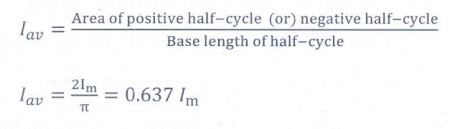14. How will you define RMS value of an alternating current?

The root mean square value of an alternating current is defined as the square root of the mean of the squares of all currents over one cycle. It is denoted by IRMS.IRMS = ŌłÜ [(Area of one cycle of squard wave) / Base length of one cycle ]   (or)

RMS value is defined as that value of the steady current which when flowing through a given circuit for a given time produces the same amount of heat as produced by the alternating current when flowing through the same circuit for the same time.

rms vm  / ŌłÜ2 = 0.707 Vm

Irms Im / ŌłÜ2  = 0.707 Im15. What are phasors?

A sinusoidal alternating voltage (or current) can be represented by a vector which rotates about the origin in anti-clockwise direction at a constant angular velocity Žē. Such a rotating vector is called a phasor.

16. Define electric resonance.

When the frequency of the applied alternating source (Žēr) is equal to the natural frequency [1/ŌłÜLC] of the RLC circuit, the current in the circuit reaches its maximum value. Then the circuit is said to be in electrical resonance.

17. What do you mean by resonant frequency?

Resonance frequency is defined as the frequency at which the impedance of the RLC circuit becomes minimum or current in the circuit becomes maximum.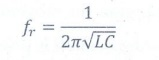18. How will you define Q-factor?

Q-factor is defined as the ratio of voltage across L or C to the applied voltage.19. What is meant by wattles current?

The current in an AC circuit is said to be wattless current if the power consumed by it is zero. This wattless current happens in a purely inductive or capacitive circuit.

20. Give any one definition of power factor.

The power factor of a circuit is defined in one of the following ways:

(i) Power factor = cos ŽĢ = cosine of the angle of lead or lag

(ii) Power factor = R/Z =         Resistance / Impedence

(iii) Power factor = VI cos ŽĢ / VI = True power / Apparent power

The power factor is defined as ratio of ture power to apparent power.

21. What are LC oscillations?

Whenever energy is given to a circuit containing a pure inductor of inductance L and a capacitor of capacitance C, the energy oscillates back and forth between the magnetic field of the inductor and the electric field of the capacitor. Thus the electrical oscillations of definite frequency are generated. These oscillations are called LC oscillations.

1. Establish the fact that the relative motion between the coil and the magnet induces an emf in the coil of a closed circuit.2. Give an illustration of determining direction of induced current by using LenzŌĆÖs law.3. Show that LenzŌĆÖs law is in accordance with the law of conservation of energy.4.     Obtain an expression for motional emf from Lorentz force.5. Give the uses of Foucault current.6. Define self-inductance of a coil interms of (i) magnetic flux and (ii) induced emf.7. How will you define the unit of inductance?8. What do you understand by self-inductance of a coil? Give its physical significance.9. Assuming that the length of the solenoid is large when compared to its diameter, find the equation for its inductance.10. An inductor of inductance L carries an electric current i. How much energy is stored while establishing the current in it?11. Show that the mutual inductance between a pair of coils is same (M12 = M21).12. How will you induce an emf by changing the area enclosed by the coil?13. Show mathematically that the rotation of a coil in a magnetic field over one rotation induces an alternating emf of one cycle.

or

Obtain the expression for the induced emf by changing relative oreientation of the coil with the magnetic field.14. Elaborate the standard construction details of AC generator.15. Explain the working of a single-phase AC generator with necessary diagram.16. How are the three different emfs generated in a three-phase AC generator? Show the graphical representation of these three emfs.17. Explain the construction and working of transformer.18. Mention the various energy losses in a transformer.19. Give the advantage of AC in long distance power transmission with an example/ilustration.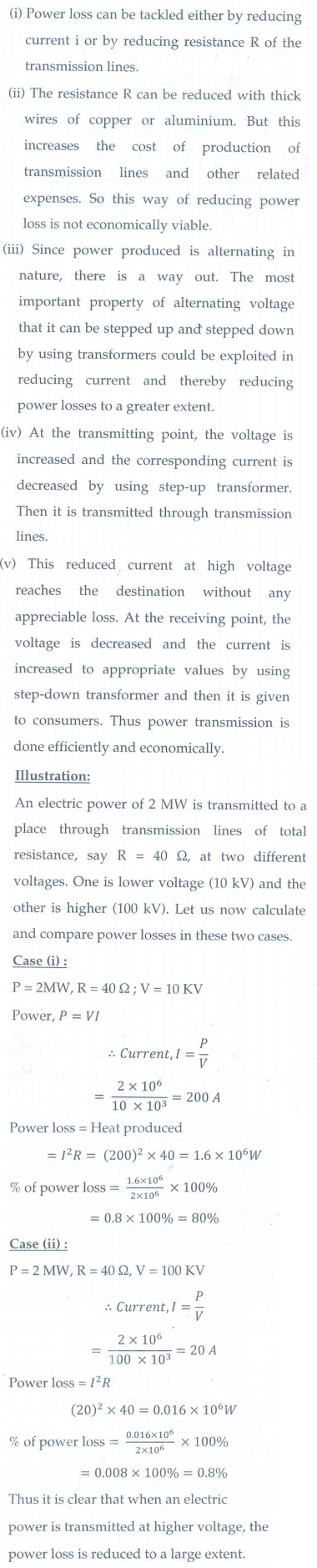20. Find out the phase relationship between voltage and current in a pure inductive circuit.21. Derive an expression for phase angle between the applied voltage and current in a series RLC circuit.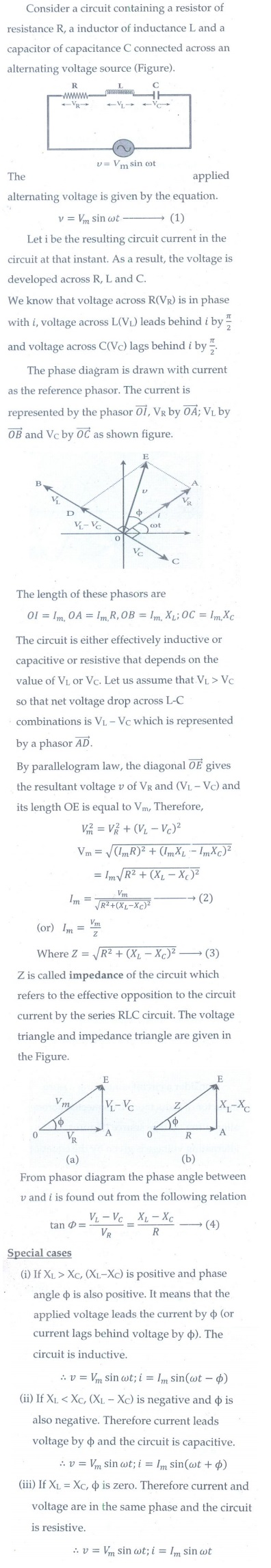22. Define inductive and capacitive reactance. Give their units.23. Obtain an expression for average power of AC over a cycle. Discuss its special cases.24. Explain the generation of LC oscillations in a circuit containing an inductor of inductance L and a capacitor of capacitance C.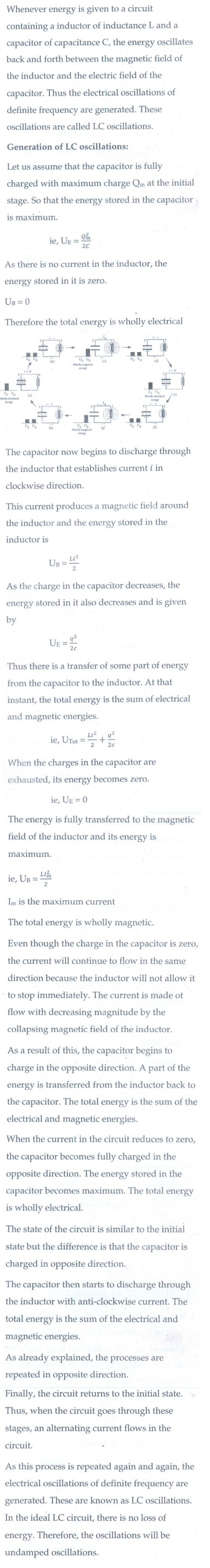25. Show that the total energy is conserved during LC oscillations.26. Compare the electromagnetic oscillations of LC circuit with the mechanical oscillations of block-spring system to find the expression for angular frequency of LC oscillators mathematically.Tags : Brief, Short Answers | Physics , 12th Physics : Electromagnetic Induction and Alternating Current
Study Material, Lecturing Notes, Assignment, Reference, Wiki description explanation, brief detail
12th Physics : Electromagnetic Induction and Alternating Current : Electromagnetic Induction and Alternating Current: Questions and Answers | Brief, Short Answers | Physics